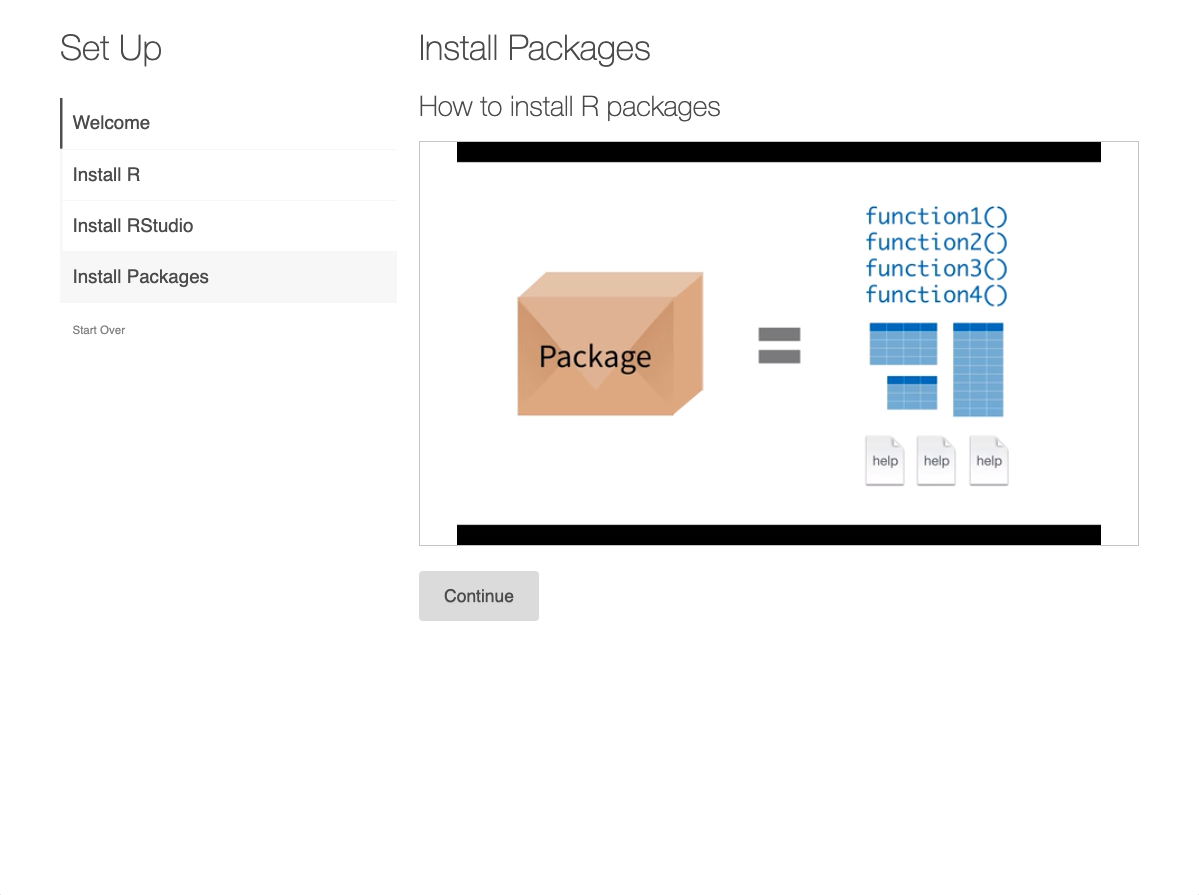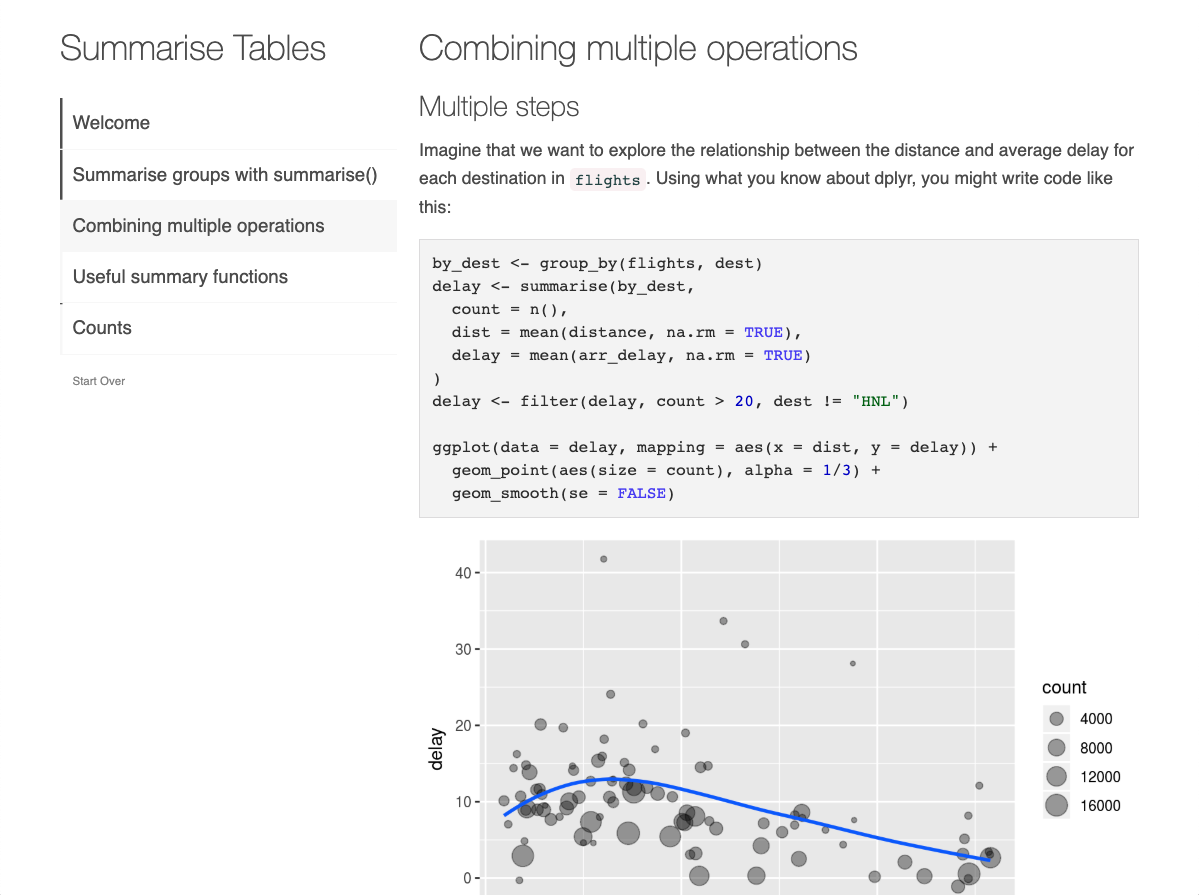The learnr package makes it easy to turn any R Markdown document into an interactive tutorial. Tutorials consist of content along with interactive components for checking and reinforcing understanding. Tutorials can include any or all of the following:

1. Narrative, figures, illustrations, and equations.

2. Code exercises (R code chunks that users can edit and execute directly).

3. Quiz questions.

4. Videos (supported services include YouTube and Vimeo).

5. Interactive Shiny components.

Tutorials automatically preserve work done within them, so if a user works on a few exercises or questions and returns to the tutorial later they can pick up right where they left off.

Examples

Here are some examples of tutorials created with the learnr package.Setting Up R

A tutorial featuring videos and interactive questions to guide a new R user through the installation and set up of everything they’ll need to get started with R.Filtering Observations

An example tutorial teaching a common data transformation: filtering rows of a data frame with dplyr::filter().Summarizing Data

An example tutorial where learners are introduced to dplyr::summarise(). Along the way, learners also gain practice with the pipe operator, %>%, and dplyr::group_by().

Hello, Tutorial!

To create a tutorial, set runtime: shiny_prerendered in the YAML frontmatter of your .Rmd file to turn your R Markdown document into an interactive app.

Then, call library(learnr) within your Rmd file to activate tutorial mode, and use the exercise = TRUE chunk option to turn code chunks into exercises. Users can edit and execute the R code and see the results right within their browser.

For example, here’s a very simple tutorial:

---
title: "Hello, Tutorial!"
output: learnr::tutorial
runtime: shiny_prerendered
---

{r setup, include=FALSE}
library(learnr)


This code computes the answer to one plus one, change it so it computes two plus two:

{r addition, exercise=TRUE}
1 + 1


This is what the running tutorial document looks like after the user has entered their answer: